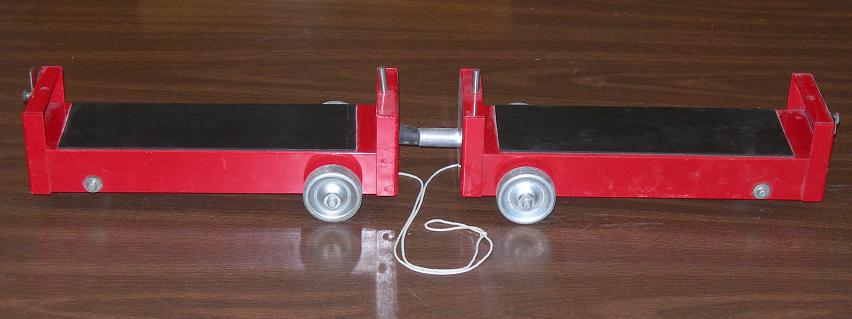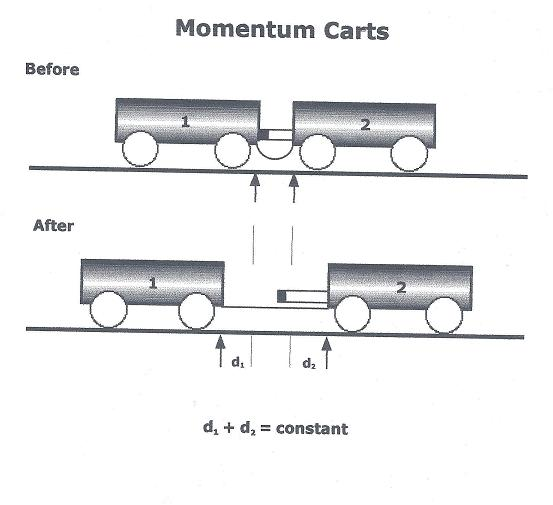Cycle II Regents Physics
John Dewey High School
Mr. Klimetz
Conservation of Momentum
An Exercise in the Application and Demonstration of the
Law of Conservation of Momentum
Laboratory Exercise
How do the speeds of the fragments of a two-fragment explosion compare with their respective masses?
IntroductionThe firing of a gun is a two-fragment explosion in which the bullet goes off in one direction and the gun recoils in the opposite direction. How can the velocities of the two fragments of such an explosion be examined and related to their masses? This laboratory exercise is designed to simulate a two-fragment explosion by releasing a compressed spring between two (nearly frictionless) carts by measuring the velocities and masses of each of these carts and examining their relationship to one another.
Equipment
Low friction (momentum) cart set (with spring apparatus)
Triple beam (or electronic) balance
Four 1-kilogram masses
Meter stick
Stopwatch
One meter length of nylon cord
Small (6.0 cm x 6.0 cm) hardwood blockProcedure
1.   To produce a simulated explosion, place the two carts in the middle of the table (Figure 1). Tie the front axle (that with two wheels) of each cart to each end of the one-meter-long nylon cord. This will ensure that neither rolls off the table. Place a 10-cm length of masking tape in the middle of the table. Draw a pencil line lengthwise down the middle. This will serve as the starting measurement line.
2.   Compress the spring in the spring-loaded cart to the first notch (low average applied force) position and lock it into place against the body of the cart. Orient the cart perpendicularly to the start line such that the far end of the rubber cushion protruding from the end of the plunger rests directly over the pencil line. Place the front of the second car agains the rubber cushion, orienting each car such that each is aligned and perpendicular to the start line. Two lab partners should be positioned respectively at the end of each of the carts. Their job is to stop each cart in place once the connecting cord has reached full extension after the spring plunger has been tripped and the carts set in motion. At this stage, the carts should be unladen (no added mass).
3.   With the small hardwood block in one hand and the stopwatch in the other, strike the trip release of the spring sharply with the block while simultaneously starting the stopwatch. Once the connecting cord has reached full extension stop the stopwatch and record. This is the time interval (t) over which the carts have traveled.
4.   While the carts are in their final positions, measure the distance from the start line to the front of each cart. This is the distance (d) over which each cart traveled. Divide d by t to calculate velocity (v). Quantitatively compare the magnitudes of the respective velocities of the two carts.
5.   Repeat steps 2 through 5 above while adding 1 kilogram to a single cart, then 2 kilograms to a single cart and then 3 kilograms to a single cart and record. Let ma be the mass of the first cart, mb be the mass of the second cart, va be the velocity of the first cart, and vb be the velocity of the second cart.
Data Processing
Calculate the momentum of each respective cart both before and after the spring is released. The momentum of car A is mava and the momentum of car B is mbvb. According to the Law of Conservation of Momentum, the sum of the momenta of each cart before the "explosion" will be the same as the sum of the momenta of each car after the "explosion". (By "explosion" we mean the tripping of the compressed spring plunger.) Since neither car is moving before the explosion, the sum of the momenta of cart A and cart B is zero. Following the explosion, the sum of the momenta of cart A and cart B should again be zero, according to the Law of Conservation of Momentum. This law predicts that the velocities of the two carts will vary inversely with their masses. The purpose of this lab is to verify this relationship.
Data Analysis
Questions
1.   Do the measured momenta of the two carts conform to the Law of Conservation of Momentum? If they do not, take the average momentum of the two carts as the best value of the momentum of each and determine the percent difference between the momenta of the two carts.
2.   Does va/vb = mb/ma as predicted? If these ratios are not nearly equal, account for the difference between them.
3.  If both carts had exactly the same mass, how would you expect their velocities to compare with each other? Explain.
4.  In the two-fragment explosion that occurs when a gun is fired, explain why the bullet acquires a high velocity and the gun does not.
5.  When the spring plunger between the carts is released, the rod pushes against the cart with a given force. This cart pushes back with an equal force. Explain why this means that the total (net) force on the system of the two carts is zero.
6.   Based on your data, calculate the impulse imparted to each cart.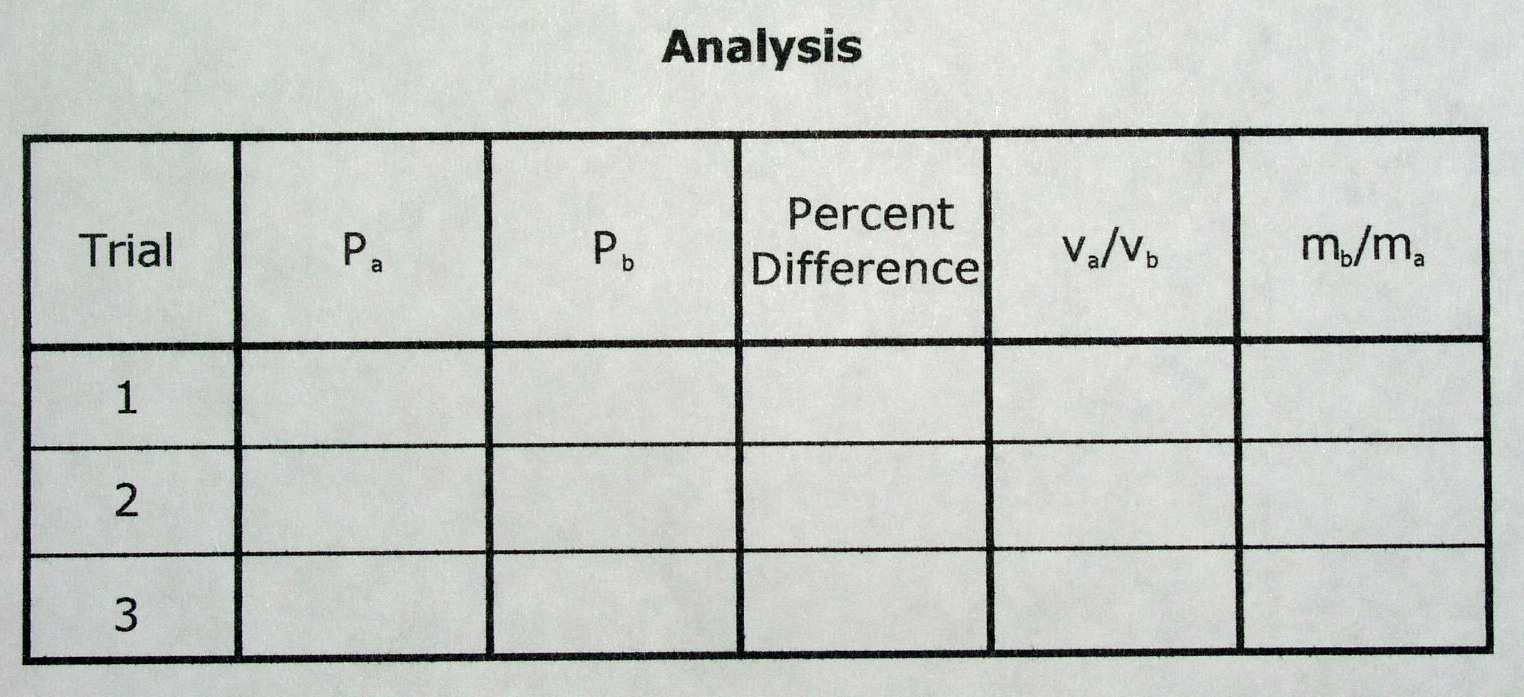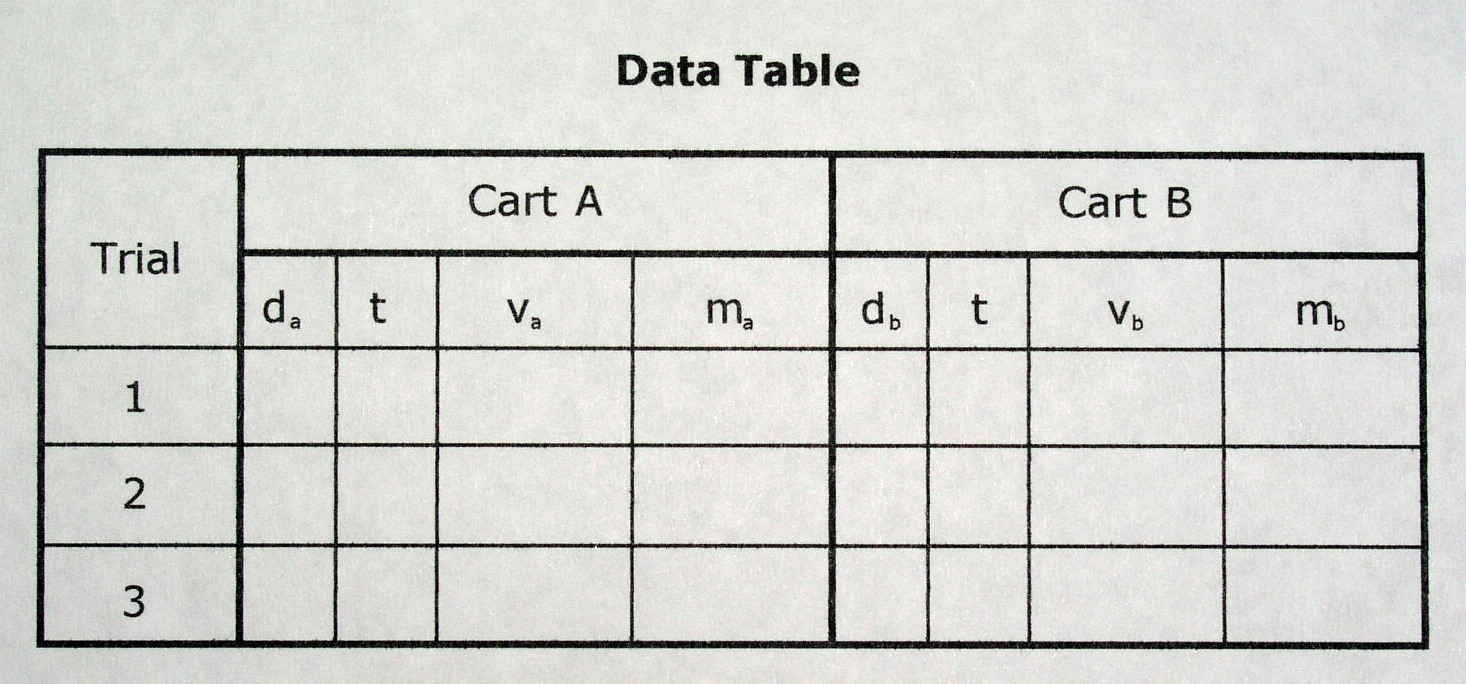Data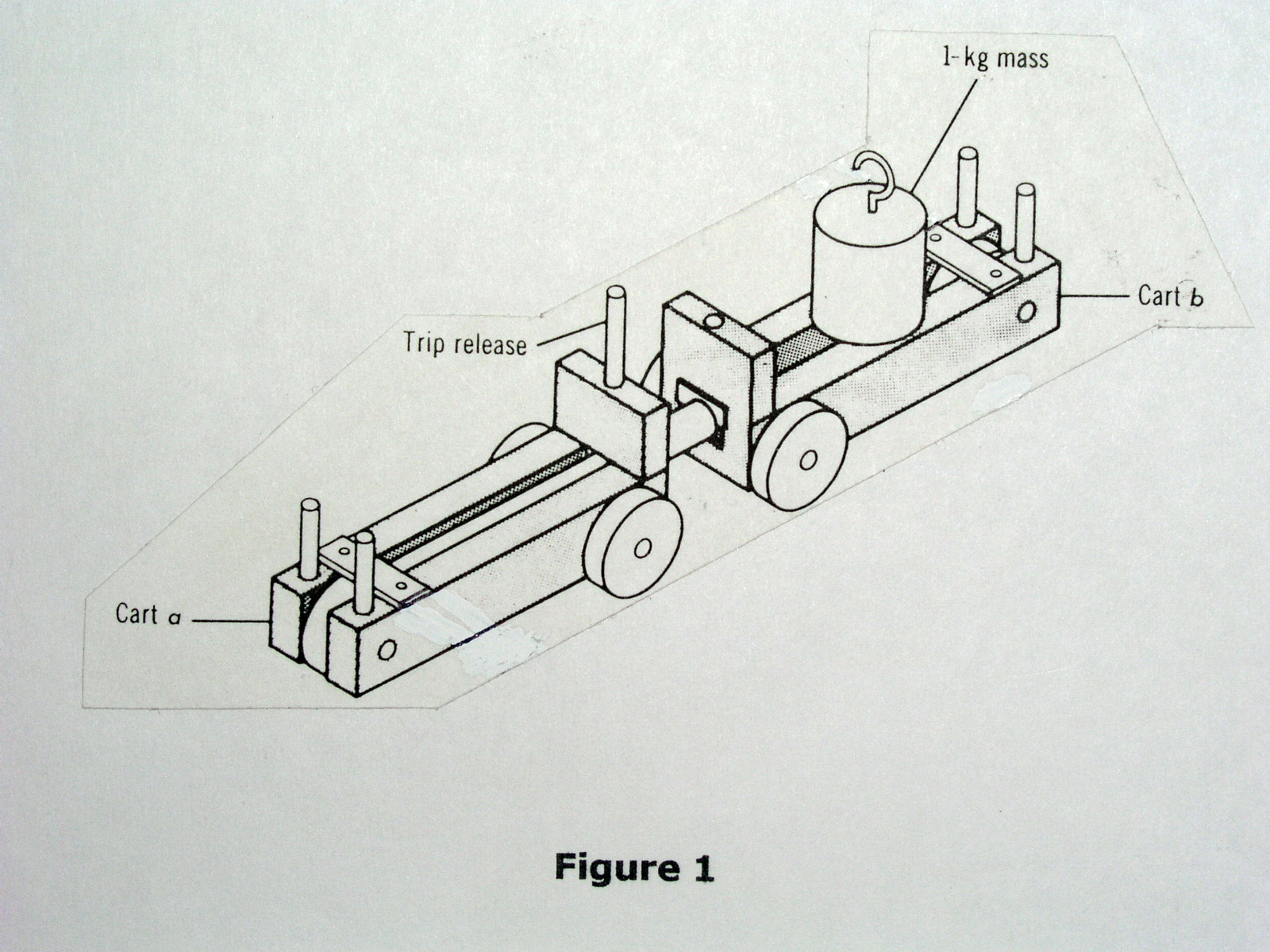Assembled ApparatusCart A
Cart B
Trip Release
1-kg MassLow-Friction Cart
Low-Friction Cart
Plunger